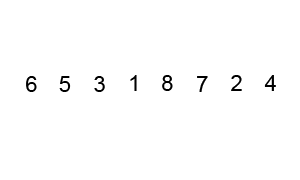Categories

## Insert-sort algorithm explained with code and dance

Insert sort is a fast algorithm for small lists. On some languages, insert sort is used to sort small arrays (small sometimes means less than 10) instead of more complex algorithms because it’s fast enough and doesn’t need much memory.To perform Insert-sort: take one element from the list on each iteration, starting from the first, and compare with the next element. If the previous one is greater, move it one position ahead. Continue comparing the rest of the elements pushing them forward until you find a the place of the current value and no elements are left. Repeat with next element in list.

O(n2)

### Pseudo code

``````function insert_sort(list):
(evaluate the list forward)
for i from 1 to length_of_list - 1:
value = list[i]
(evaluate the list backward from i):
while prev_position >= 0 and list[prev_position] > value:
(swap values from current position to the next)
list[prev_position+1] = list[prev_position]
go on position back in the list
if there was no swap, set value back in current position
``````

### PHP code example

``````function insert_sort(\$list) {
for(\$i = 0; \$i < count(\$list); \$i++) {
\$value = \$list[\$i];
\$j = \$i - 1;
while(\$j >= 0 AND \$list[\$j] > \$value) {
\$list[\$j + 1] = \$list[\$j];
\$j--;
}
\$list[\$j + 1] = \$value;
}
return \$list;
}
``````

### Python code example

``````def insert_sort(unordered_list):
for i in xrange(0,len(unordered_list)):
value = unordered_list[i]
j = i -1
while (j >= 0) and (unordered_list[j] > value):
unordered_list[j+1] = unordered_list[j]
j -= 1
unordered_list[j+1] = value
return unordered_list
``````

Insert sort algorithm can be explained better visually with a Romanian folk dance:

Categories

## Basic programming algorithm: Bubble Sort

Bubble sort is one of the most basic sorting algorithms in taught in computer science classes. It will order values in a list from smallest to largest passing several times through the list comparing two items at a time and reordering them. This algorithm is not very efficient with large lists because it has to evaluate every member of the list several times. The longer the list, the more it will take the algorithm to finish. In big-O notation, this algorithm’s efficiency is represented by: O(n2)

An explanation of bubble sort from Wikipedia:

Bubble sort, sometimes incorrectly referred to as sinking sort, is a simple sorting algorithm that works by repeatedly stepping through the list to be sorted, comparing each pair of adjacent items and swapping them if they are in the wrong order. The pass through the list is repeated until no swaps are needed, which indicates that the list is sorted. The algorithm gets its name from the way smaller elements “bubble” to the top of the list. Because it only uses comparisons to operate on elements, it is a comparison sort. Although the algorithm is simple, most of the other sorting algorithms are more efficient for large lists.While passing through the list, compare two elements, if the second one is bigger than the first, swap their positions in the list. Continue going through the list, comparing the next elements in the same way. Go through the list until no more swaps take place.

Pseudocode:

``````while not sorted:
sorted = True
for iterator = 1 to length_of_list - 1 do:
check if pair is out of order:
if list[iterator] is bigger than list[iterator+1] then
swap them:
temporal_value = list[iterator]
list[iterator] = list[iterator+1]
list[iterator+1] = temporal_value
sorted = False
``````

PHP code example:

``````/**
* Sort an array using bubble sort algorithm
* @param array \$list The list to be sorted
* @return array The sorted array
*/
function bubbleSort(\$list) {
while(!\$sorted){
\$sorted = True;
foreach(\$list as \$key => \$value) {
if(\$list[\$key] > \$list[\$key+1]) {
\$temp = \$list[\$key+1];
\$list[\$key+1] = \$list[\$key];
\$list[\$key] = \$temp;
\$sorted = False;
}
}
}
return \$list;
}
``````

Python code example:

``````function bubbleSort(a_list):
""" Sort an array using bubble sort algorithm """
sorted = False
length = len(a_list)
while not sorted:
sorted = True
for position in range(length):
if a_list[position] > a_list[position+1]:
# Swap values
a_list[position], a_list[position+1] = a_list[position+1], a_list[position]
sorted = False
return a_list
``````

There is another way to write the bubble sort, by shrinking the list by one element after every pass. If you see the animated graphic above, after each pass, the last number is already in its correct place, so you don’t have to iterate over those every subsequent time. You can discard the last element each time, making the algorithm faster.

An example of this version in Python code would look like this:

``````function betterBubbleSort(a_list):
""" Sort an array using bubble sort algorithm """
length = len(a_list)
while length:
for position in range(length):
if a_list[position] > a_list[position+1]:
# Swap values
a_list[position], a_list[position+1] = a_list[position+1],a_list[position]
length -= 1
return a_list
``````

Do you have other ways of writing the bubble sort? Share them in the comments section below.